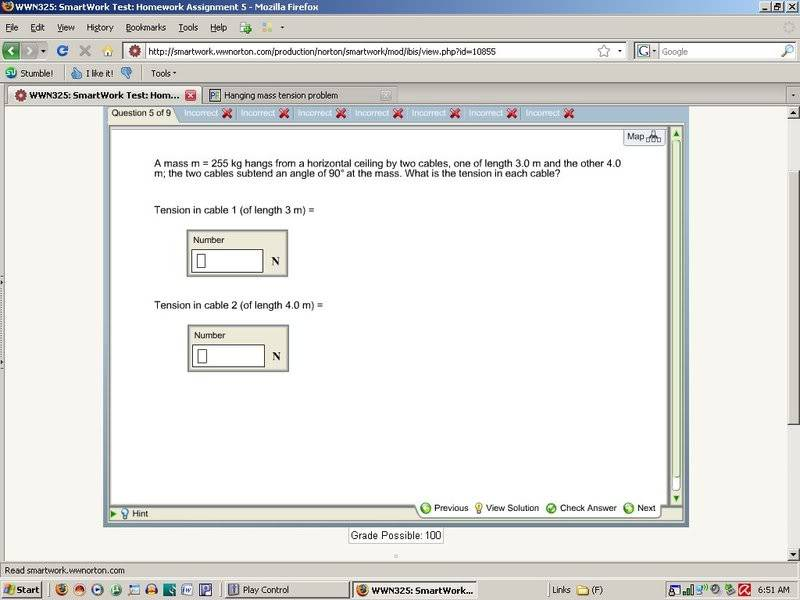# Hanging mass tension problem

## Homework Statement

A mass (m=255kg) hangs from a horizontal ceiling by two cables, one of length 3m and the other 4m. The two cables subtend an angle of 90 degrees at the mass. What is the tension in each cable?

F=ma
T=ma?

## The Attempt at a Solution

It's in equilibrium, so the forces sum to zero. F=ma so, 255kg(9.81)? or 255kg(-9.81)? I am confused about the word subtend? I know a chord in a circle subtends the arc... but here..? The lengths of the cables are confusing me, how can the ceiling be horizontal, and the cables make 90 degree angles with the mass, but be different lengths? So I must be interpreting the information wrong and making it harder then it is. Ah!

## Answers and Replies

That is quite poorly worded, is there a diagram with the example?

I have interpreted it as in the image below:

http://img519.imageshack.us/img519/7097/subtendyd7.png [Broken]

So use trigonometry to calculate the two angles that meet the ceiling.
Then you can create a simultaneous equation. You are correct that it's in equilibrium so sum of forces = 0

Using this, let A = tension in rope of length 3, and B = tension in rope of length 4
Acos(z) - Bcos(x) = 0 (1)
where z is the angle A makes with the roof and x is the angle B makes with the roof.

Also, the weight of the mass creates a force of F = mg
therefore Asin(z) + Bsin(x) = -mg (2)

The positive and negative sign of mg does not matter, as long as the two equal forces have opposite signs such that the sum of all forces = 0.

Solve the two simultaneous equations (1) and (2) for A and B and voila!

Last edited by a moderator:Terrible presentation. They should always give a diagram in examples such as this. If I were you, I would follow the instructions I gave in the previous post.
It seems to be the most logical conclusion to me.

Code:
Using this, let A = tension in rope of length 3, and B = tension in rope of length 4
Acos(z) - Bcos(x) = 0 (1)
where z is the angle A makes with the roof and x is the angle B makes with the roof.

Also, the weight of the mass creates a force of F = mg
therefore Asin(z) + Bsin(x) = -mg (2)

The positive and negative sign of mg does not matter, as long as the two equal forces have opposite signs such that the sum of all forces = 0.

Solve the two simultaneous equations (1) and (2) for A and B and voila!

...so

cos(z)= 53.1301 degrees
cos(x)= 36.8699 degrees

(1) A53.1301 - B36.8699 = 0 solve for A

A= .693955B

then..

sin(z)= .8
sin(x)= .6
-mg= 2501.55 because --> 255kg(9.81)

(2) A.8 + B.6 = -2501.55 substitute in A= .693955B

I get B= -2165.54

So I go back to eq. (1) and substitute in B= -2165.54 and I get

A= -1502.79

A and B are the tensions of cables length 3 and 4 but I did something wrong. Did I approach this completely backwards or what? I think I'm making it more difficult then it is.

Code:
 [...]

cos(z)= 53.1301 degrees
cos(x)= 36.8699 degrees

[B][U](1) [B]A53.1301 - B36.8699 = 0[/B] solve for A[/U][/B]

A= .693955B

[...][/QUOTE]

Read over my instructions again. You forgot some sines and cosines

cos(z) does not equal 53 degrees. z = 53 degrees.
same with cos(x).

so Acos(53) - Bcos(37) = 0
that's your first equation.

I appreciate your help! I did not solve this yet, I'll be back later, I actually have to run to physics class right now.

I appreciate your help! I did not solve this yet, I'll be back later, I actually have to run to physics class right now.

I can't see why you think you've done something wrong. I solved the equation just then and got the tension in the 3m rope to be 1997N and in the 4m rope to be 1505N. These are of the same order of your answers, so some simple significant figures could change a lot.